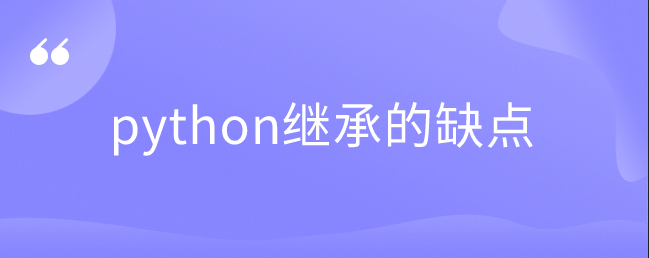# python继承的缺点小妮浅浅2021-09-09 11:42:39原创1341、可能特殊的类存在其他特殊的地方，定义一个类，其下也可以重新定义类，这样会导致继承的那条线越来越长。

2、如果使用继承，任何一点小小的变化都需要重新定义一个类，很容易导致类别的爆炸式增长。

```class SubDict(dict):
def __setitem__(self, k, v):
v = "sub_item_"+str(v)
super().__setitem__(k, v)

sd = SubDict(one="one")
print(sd)
sd['two']='two'
print(sd)
sd.update(three="three")
print(sd)
# {'one': 'one'}
# {'one': 'one', 'two': 'sub_item_two'}
# {'one': 'one', 'two': 'sub_item_two', 'three': 'three'}```